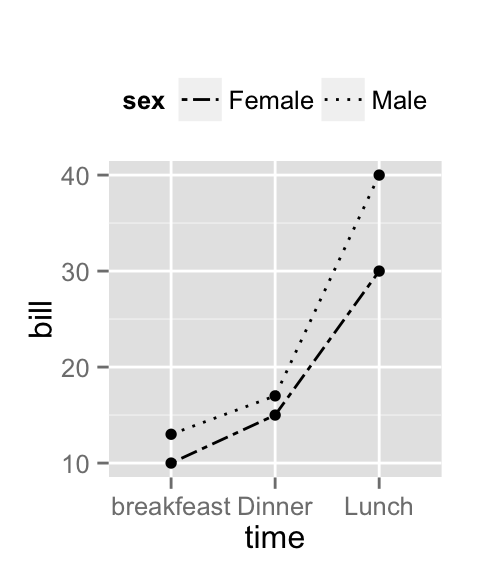# ggplot2 line types : How to change line types of a graph in R software?

This R tutorial describes how to change line types of a graph generated using ggplot2 package.

# Line types in R

The different line types available in R software are : “blank”, “solid”, “dashed”, “dotted”, “dotdash”, “longdash”, “twodash”.

Note that, line types can be also specified using numbers : 0, 1, 2, 3, 4, 5, 6. 0 is for “blank”, 1 is for “solid”, 2 is for “dashed”, ….

A graph of the different line types is shown below :# Basic line plots

## Generate some data

``````df <- data.frame(time=c("breakfeast", "Lunch", "Dinner"),
bill=c(10, 30, 15))
``````##         time bill
## 1 breakfeast   10
## 2      Lunch   30
## 3     Dinner   15``````

## Create line plots and change line types

The argument linetype is used to change the line type :

``````library(ggplot2)
# Basic line plot with points
ggplot(data=df, aes(x=time, y=bill, group=1)) +
geom_line()+
geom_point()
# Change the line type
ggplot(data=df, aes(x=time, y=bill, group=1)) +
geom_line(linetype = "dashed")+
geom_point()``````# Line plot with multiple groups

## Create some data

``````df2 <- data.frame(sex = rep(c("Female", "Male"), each=3),
time=c("breakfeast", "Lunch", "Dinner"),
bill=c(10, 30, 15, 13, 40, 17) )
``````##      sex       time bill
## 1 Female breakfeast   10
## 2 Female      Lunch   30
## 3 Female     Dinner   15
## 4   Male breakfeast   13
## 5   Male      Lunch   40
## 6   Male     Dinner   17``````

## Change globally the appearance of lines

In the graphs below, line types, colors and sizes are the same for the two groups :

``````library(ggplot2)
# Line plot with multiple groups
ggplot(data=df2, aes(x=time, y=bill, group=sex)) +
geom_line()+
geom_point()
# Change line types
ggplot(data=df2, aes(x=time, y=bill, group=sex)) +
geom_line(linetype="dashed")+
geom_point()
# Change line colors and sizes
ggplot(data=df2, aes(x=time, y=bill, group=sex)) +
geom_line(linetype="dotted", color="red", size=2)+
geom_point(color="blue", size=3)``````## Change automatically the line types by groups

In the graphs below, line types, colors and sizes are changed automatically by the levels of the variable sex :

``````# Change line types by groups (sex)
ggplot(df2, aes(x=time, y=bill, group=sex)) +
geom_line(aes(linetype=sex))+
geom_point()+
theme(legend.position="top")
# Change line types + colors
ggplot(df2, aes(x=time, y=bill, group=sex)) +
geom_line(aes(linetype=sex, color=sex))+
geom_point(aes(color=sex))+
theme(legend.position="top")``````## Change manually the appearance of lines

The functions below can be used :

• scale_linetype_manual() : to change line types
• scale_color_manual() : to change line colors
• scale_size_manual() : to change the size of lines
``````# Set line types manually
ggplot(df2, aes(x=time, y=bill, group=sex)) +
geom_line(aes(linetype=sex))+
geom_point()+
scale_linetype_manual(values=c("twodash", "dotted"))+
theme(legend.position="top")
# Change line colors and sizes
ggplot(df2, aes(x=time, y=bill, group=sex)) +
geom_line(aes(linetype=sex, color=sex, size=sex))+
geom_point()+
scale_linetype_manual(values=c("twodash", "dotted"))+
scale_color_manual(values=c('#999999','#E69F00'))+
scale_size_manual(values=c(1, 1.5))+
theme(legend.position="top")``````# Infos

This analysis has been performed using R software (ver. 3.1.2) and ggplot2 (ver. 1.0.0)